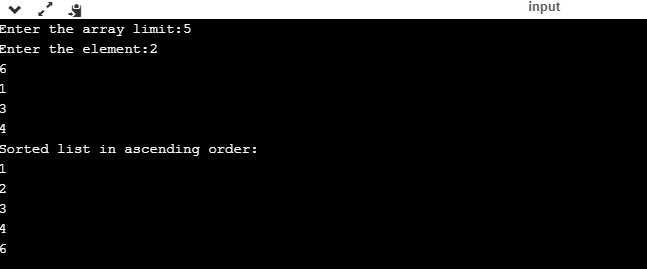UncleCoder.com

UncleCoder.com

Free programming examples and instructions

# C Program to study Bubble Sort

## C Program to study Bubble Sort

by Krishna

Posted on 27 Jul 2018 Category: c Views: 1850

C Program to study Bubble Sort

Bubble Sort is the simplest sorting algorithm that works by repeatedly swapping the adjacent elements if they are in the wrong order.
If we have total n elements, then we need to repeat this process for n-1 times. In this section, we will write a C program to perform bubble sort.

``````#include <stdio.h>

int main()
{
int array, n, c, d, swap;

printf("Enter the array limit:");
scanf("%d", &n);

printf("Enter the element:");
for (c = 0; c < n; c++)

scanf("%d", &array[c]);

for (c = 0 ; c < n - 1; c++)
{
for (d = 0 ; d < n - c - 1; d++)
{
if (array[d] > array[d+1]) /* For decreasing order use < */
{
swap       = array[d];
array[d]   = array[d+1];
array[d+1] = swap;
}
}
}

printf("Sorted list in ascending order:\n");

for (c = 0; c < n; c++)
printf("%d\n", array[c]);

return 0;
}
``````

OUTPUT### Latest posts in c

##### C program to find largest among two numbers• View all

• View all

• View all

• View all

• View all